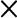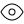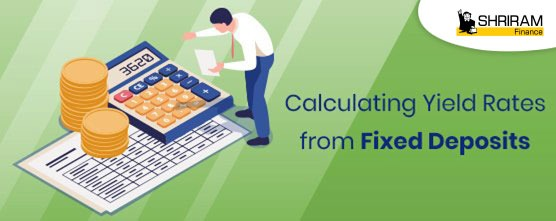Fixed Deposit up to 9.05% p.a.* Interest rates. Invest Now

01-01-2023 00:13:59

Recurring Deposit up to 8.50% p.a.* Interest rates. Invest Now

14-11-2022 14:09:00

Two Entities - One Shared Vision - STFC and SCUF are now merged as Shriram Finance

14-11-2022 14:08:45

# Calculating Yield Rates from Fixed Deposits

Posted: 23rd October, 2021

Updated: 8th February, 2023

Written by -Fixed deposits are fixed-income saving schemes. You choose the amount you want to deposit and the tenure, and depending on these factors, the fixed deposit interest rateis determined.

When you know the fixed deposit yield interest rate, you can plan for your financial goals effectively. Therefore, when you make a deposit, it is advisable to calculate the interest so that you know the corpus that you can accumulate.

But how do you do that?

It is simple. There is a formula for calculating the interest that you can earn.

But before we look at the formula, you should know that there are two types of interest calculations on fixed deposit schemes. Let us see them.

## Types of fixed deposit interest calculations

1. 1. Simple interest calculation

Under the simple interest mode of interest calculation, you receive a flat interest rate on the amount you deposit. So, if you deposit Rs. 100 and the interest rate is 6%, you would earn an interest of Rs. 6 every year.

1. 2. Compound interest calculation

Under the compound interest mode of calculation, interest is calculated each year on the corpus accumulated at the end of the last year. As a result, you earn interest not only on the deposited amount but also on the interest already added previously.

Are you confused?

Let us simplify.

In the above example, after the first year, you would earn an interest of Rs. 6 on the deposit of Rs. 100. However, in the second year, the interest would be calculated on Rs. 106, i.e., Rs. 100, which you deposited, and the interest of Rs. 6 that you earned last year. So, in the second year, the interest payable would be Rs. 6.36. Similarly, in the third year, you will calculate the interest on the total amount of Rs.106 + Rs.6.36, i.e., Rs.112.36.

## The formula for Fixed deposit yield interest rate

Now that you know the two types of interest calculations, here is a look at the formula for each:

1. 1. Simple interest = (P * R * T) / 100
2. 2. Compound interest = [P * {(1+R/100) ^ T}] - P

In the above formulae, the values used are as follows:

• P = the principal, i.e., the amount of money that you deposit
• R = the fixed deposit interest rate
• T = the term of the deposit

Let us understand with the help of a good example:

Say you deposit Rs. 50,000 in a fixed deposit scheme which pays an interest of 8% per annum for a term of 5 years. In this case, you can calculate the yield from the plan under both simple and compound interest as follows:

 Simple interest calculation Compound interest calculation Interest earned = (P * R * T) / 100 = (50,000 * 8 * 5) / 100 = Rs.20,000 Interest earned = [P * {(1+R/100) ^ T}] - P = 50,000 * {(1+8/100)^5} - 50,000 = Rs.23,466 (rounded-off)

## Using the fixed deposit calculator

While the formulae are pretty simple, and you can work them out to find the fixed deposit yield interest rate, it is a technical calculation. As such, fixed deposit calculators are available, which help you calculate the potential interest with accuracy and ease.

The fixed deposit calculator is an online tool wherein you just need to enter the amount you are depositing, the tenure of deposit, and the interest rate. The calculator then estimates the interest you can earn on the deposit and the amount payable on the maturity. You can also change the deposit amount and/or the tenure as per your needs to find how the interest earnings would change.

## Benefits of fixed deposit calculator

A fixed deposit calculator is a beneficial tool because:

1. 1. It is free of cost.
2. 2. It can give you the interest amount within seconds.
3. 3. The results are accurate and error-free.
4. 4. It is readily available.
5. 5. You can use it as many times as you want.
6. 6. It helps you compare different fixed deposit schemes so that you can choose one which pays the highest interest income on your savings.

## Things to know about fixed deposit interest rate

Fixed deposits give you guaranteed returns and are immune to volatility risks. While you can find the best fixed deposit yield interest rates, here are some aspects that you should keep in mind.

1. 1. There are two ways of availing interest- the cumulative basis and the non-cumulative basis. If you choose the first option, the interest earned would be added to your deposit amount and then paid in a lump sum on maturity. Under the second option, however, you receive the interest as and when earned Many fixed deposit schemes allow you to choose the interest payment frequency from the monthly, quarterly, half-yearly, or annual mode.
2. 2. Under most schemes of fixed deposits, compound interest is payable. You can compound the interest annually, half-yearly, or quarterly. If compounding is half-yearly or quarterly, the formula will change.
3. 3. Senior citizens usually receive a higher rate of interest.
4. 4. The interest that you earn would be taxable in your hands. However, if you are a senior citizen, interest income up to Rs. 50,000 would be tax-free.
5. 5. If you make a premature withdrawal, i.e., withdraw before the term has expired, the lender will reduce your fixed deposit interest rate

## Shriram Finance fixed deposits

Shriram Finance Fixed Deposits are awarded the highest credit rating of [ICRA]AA+ (Stable)” by ICRA and "IND AA+/Stable" by India Ratings and Research. Therefore, you rest assured about the security of your deposit. You can avail of 12 to 60 months tenure. Moreover, the Shriram Finance provides interest rate of up to 9.05%* p.a. which is inclusive of the additional interest benefit of 0.50%* p.a. for senior citizens and 0.10%* p.a. for women depositors.

You can use the Shriram Finance fixed deposit calculator to find out the maturity corpus. Just enter in the deposit amount and the tenure. The calculator would calculate the interest payable as well as the maturity amount.

You can use the Shriram Finance fixed deposit calculator to find out the maturity corpus. Just enter in the deposit amount and the tenure. The calculator would calculate the interest payable as well as the maturity amount.

Invest in Shriram Finance fixed deposits for attractive returns and choose from the company's flexible schemes.

18001034959
18001036369
02241574545